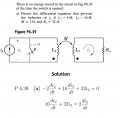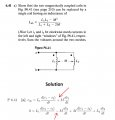# Confusing Mututal Inductance with conventioal Dots !

#### Mohamed Mokhtar

Joined Sep 30, 2015
12
Hi, i am totally confused about determining the polarity of the induced voltage by the Mutual Inductance, i know that the dots are there to determine the polarity of the iduced voltage but in these problems i can's see how it helps

the first problem :it is all about summing voltages in the loop of i2 but WHY subtracting the induced voltage ?? (Note that the Dots are in the same direction)

and the second problem made it more confusing :again summing voltages in loops , here we have the inductor L1 common in both loops , WHY on earth did he sum the induced voltage of L1 in the first loop and subtract the same induced voltage of the same inductor in the second loop (Note again the Dots are in the sam direction like the first problem)

Can someone help me understaind how these Dots determine when to sum / subtract the induced voltage ?? Thanks for your time.

#### MrAl

Joined Jun 17, 2014
10,587
Hi,

The usual convention involves two rules:
1. If the voltage that appears at the dotted terminal of one coil is positive then the voltage that appears at the dotted terminal of the second coil is also positive.
2. If the current that enters the dotted terminal of of one coil is positive then the current that enters the dotted terminal of the second coil is also positive.

Obviously a current or voltage that is opposite to the above produces an opposite effect.

One example of this is that for two coils that both have dots at the top and both have currents entering at the dots (one current circulates clockwise and the other counter clockwise) then everything is positive:
v1=L1*di1/dt+M*di2/dt
v2=L2*di2/dt+M*di1/dt

However if the current in the second coil also circulates clockwise and the polarity of v1 is flipped (negative on top), then we have:
v1=-L1*di1/dt+M*di2/dt
v2=-L2*di2/dt+M*di1/dt

So three things are involved here:
The direction of the current,
the assumed polarity of the voltage,
and the dot relative to the current and voltage.

See if that helpsWe might be able to come up with a schematic of two coils with mutual inductance by replacing them with isolated coils and adding two extra coils that emulates the mutual inductance and thereby reduce the problem to four isolated coils (and some other parts). That would probably make these questions a lot simpler.

Last edited:

#### hsazerty2

Joined Sep 25, 2015
30
To sum the induced voltage, both currents of the inductors must enter or exit the dots, at the same time. (second problem)

Otherwise you sustract.(first problem)